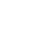# Rammstein Boots

•••••\$140.00
In stock.

10 hole "RAMMSTEIN" leather boots with logo embroidery on the outer side, with stable screwed, sewn and glued rubber sole and steelcaps in the toes of the boots.

Material: 100% leather, rubber sole.

Bootleg height: 18 cm.

Please do NOT use the box as a shipping box for returns.

UK - EU - US

3 = 36 = 5
3.5 = 37 = 5.5

4 = 38 = 6
5 = 39 = 7
6 = 40 =7.5
7 = 41 = 8.5
8 = 42 = 9
9 = 43 =10
10 = 44 = 10.5
11 = 45 = 11.5
12 = 46 = 12
13 = 47 = 13

RAMMSTEINSHOP RECOMMENDS